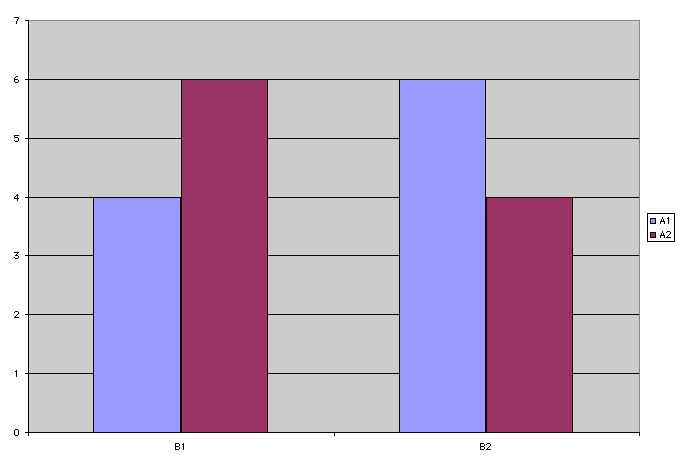# Test Yourself

Take this quiz and see how you do. Select the correct answers by using the drop down menus. Once you are done, click submit to see your score! The ones that you get wrong will have a check!

 A1 A2 B1 4 6 B2 5 7

1. In the table above, is there a main effect for A?
2. In the table above, is there a main effect for B?
3. In the table above, is there an interaction?4. In the figure above, is there a main effect for A?
5. In the figure above, is there a main effect for B?
6. In the figure above, is there an interaction?
7. If the total df in a factorial experiment is 14, there are ____ participants.
8. If the df for Treatment A is 2, there are ____ levels of Treatment A.
9. If the df for Treatment A is 1 and the df for Treatment 4, the df for the A X B interaction is

You got out of correct.